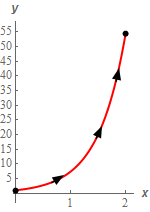Paul's Online Notes
Home / Calculus III / Line Integrals / Line Integrals - Part II
Show Mobile Notice Show All Notes Hide All Notes
Mobile Notice
You appear to be on a device with a "narrow" screen width (i.e. you are probably on a mobile phone). Due to the nature of the mathematics on this site it is best views in landscape mode. If your device is not in landscape mode many of the equations will run off the side of your device (should be able to scroll to see them) and some of the menu items will be cut off due to the narrow screen width.

### Section 16.3 : Line Integrals - Part II

1. Evaluate $$\displaystyle \int\limits_{C}{{\sqrt {1 + y} \,dy}}$$ where $$C$$ is the portion of $$y = {{\bf{e}}^{2x}}$$ from $$x = 0$$ to $$x = 2$$.

Show All Steps Hide All Steps

Start Solution

Here is a quick sketch of $$C$$ with the direction specified in the problem statement shown.Show Step 2

Next, we need to parameterize the curve.

$\vec r\left( t \right) = \left\langle {t,{{\bf{e}}^{2t}}} \right\rangle \hspace{0.25in}0 \le t \le 2$ Show Step 3

Now we need to evaluate the line integral. Be careful with this type line integral. Note that the differential, in this case, is $$dy$$ and not $$ds$$ as they were in the previous section.

All we need to do is recall that $$dy = y'\,dt$$ when we convert the line integral into a “standard” integral.

So, let’s evaluate the line integral. Just remember to “plug in” the parameterization into the integrand (i.e. replace the $$x$$ and $$y$$ in the integrand with the $$x$$ and $$y$$ components of the parameterization) and to convert the differential properly.

Here is the line integral.

\begin{align*}\int\limits_{C}{{\sqrt {1 + y} \,dy}} &= \int_{0}^{2}{{\sqrt {1 + {{\bf{e}}^{2t}}} \,\,\left( {2{{\bf{e}}^{2t}}} \right)\,dt}}\\ & = \left. {\left[ {\frac{2}{3}{{\left( {1 + {{\bf{e}}^{2t}}} \right)}^{\frac{3}{2}}}} \right]} \right|_0^2 = \require{bbox} \bbox[2pt,border:1px solid black]{{\frac{2}{3}\left[ {{{\left( {1 + {{\bf{e}}^4}} \right)}^{\frac{3}{2}}} - {2^{\frac{3}{2}}}} \right] = 274.4897}}\end{align*}

Note that, in this case, the integral ended up being a simple substitution.